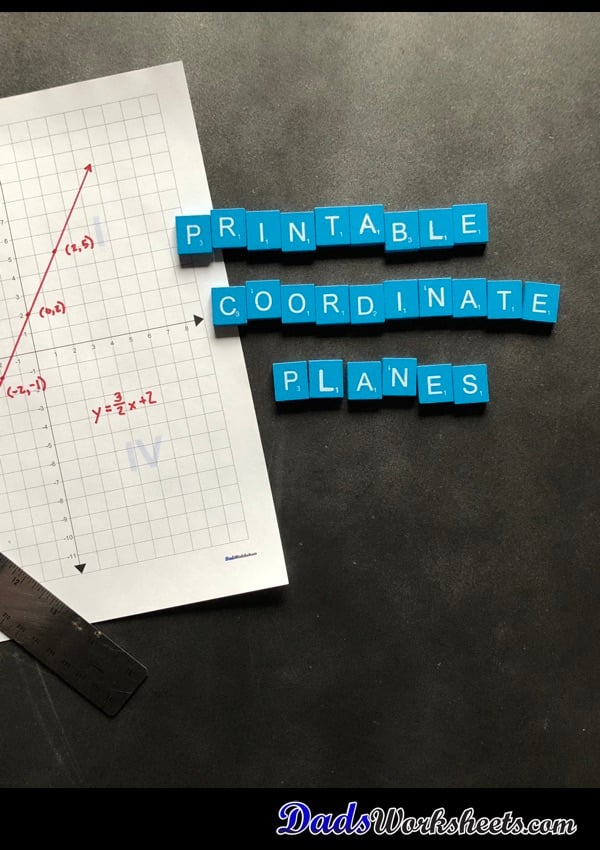# Coordinate Plane

The blank coordinate planes on this page include variations with labels on either the axis or the edge of the grid, as well as versions with quadrant labels. You can find full 4 quadrant coordinate planes, as well as just blank 1 quadrant coordinate planes in layouts setup for solving multiple homework problems on a single page.

### 8 Coordinate Plane Worksheets

These printable coordinate planes have each quadrant labeled in lighter background text in the grid. Confused with all those I, II, II, IV Roman numeral labels? You'll know where they are.

## Blank Coordinate Plane Work Pages

### 28 Coordinate Plane Worksheets

Print out these blank coordinate pages with name and date blocks when you've got equations to graph for homework! The homework you turn in for geometry or algebra class will look academically astute.

Blank Coordinate Plane Work Pages

## Coordinate Plane Without Labels

### 8 Coordinate Plane Worksheets

Printable coordinate planes in inch and metric dimensions in multiple sizes, great for scatterplots, plotting equations, geometry problems or other similar math problems. These are full four-quadrant coordinate planes, blank without axis numbering. Flashing back to 7th grade geometry class? This is the graph paper page for you. Standard sizes 1/4 inch, 1/5 inch, 1/8 inch and 1/10 inch grids . Metric sizes 1 centimeter, 5 millimeter, 2.5 millimeter and 2 millimeter grids.

Coordinate Plane Without Labels

## Coordinate Plane With Labeled Axis

### 8 Coordinate Plane Worksheets

Printable coordinate planes in inch and metric dimensions in various sizes, great for plotting equations, geometry problems or other similar math problems. These are full four-quadrant graphs. These coordinate planes have labels directly along the x axis and y axis.

Coordinate Plane With Labeled Axis

## Coordinate Plane With Labeled Edges

### 8 Coordinate Plane Worksheets

Printable coordinate planes in inch and metric dimensions in various sizes, great for plotting equations, geometry problems or other similar math problems. These are full four-quadrant graphs. These coordinate planes have x axis and y axis labels along the outer edge of the page.

Coordinate Plane With Labeled Edges

### 24 Coordinate Plane Worksheets

This printable coordinate plane with quadrant 1 only shown is great for introducing graphing activities in 3rd, 4th or 5th grade, or any time before negative numbers are introduced.

## Printable Blank Coordinate Plane Pages

The collection of printable PDF coordinate planes on this page provide a number of different layouts that should fit the needs of most middle school or high school algebra classes. The coordinate planes are dimensioned in customary or metric units, just like the blank graph paper on the site.

There are versions of the coordinate plane with and without axis labels, as well as versions that show the quadrant numbers in light text in the background of each quadrant. There are also versions of the coordinate plane with labels on the edges instead of on each axis itself, which can sometimes make graphing equations a little easier.

### What are Coordinate Plane Quadrants?

Because a coordinate plane is naturally divided by its x axis and y axis, it creates four rectangular regions that are called quadrants. Each quadrant corresponds to a region containing points with the same positive or negative sign. For example, by convention the upper right quadrant is called quadrant 1 and contains only points that have both positive x and positive y values. The breakdown of the signs of coordinate points and their corresponding quadrants is shown in the table below...